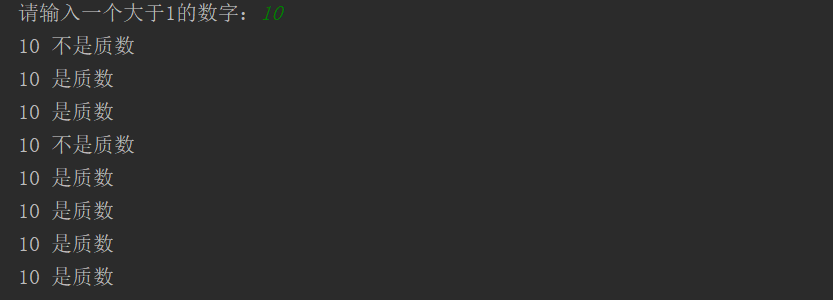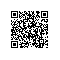# 水仙花数以及质数的判断 | Python从入门到精通：入门篇之十六

i=0
result=0
while i<100:
i+=1
if i % 2 != 0 :
print(i)
result+=i
print('result=：',result)

i=0
result=0
count=0
while i < 100 :
i+=1
if i % 7 ==0 :
print(i)
result+=i
count+=1
print('100以内7的倍数之和:',result)
print('100以内7的倍数的个数：',count)

n=100
while n<1000 :
a=n//100 #百位数字
b=(n%100)//10 #十位数字
c=n%10 #个位数字
if a**3+b**3+c**3==n :
print(n)
n+=1 #更新while循环的条件

n=int(input('请输入一个大于1的数字：'))
i=2
while i<n :
# 如果n能够被i整除，则n不是质数
if n % i ==0 :
print(n, '不是质数')
else:
print(n, '是质数')
i+=1n=int(input('请输入一个大于1的数字：'))
i=2
flag=True
while i<n :
#如果n能够被i整除，则n不是质数
if n % i ==0 :
#不是质数则改变flag的值
flag=False
i+=1 #i为2到n的所有的值。
#循环结束之后，如果flag为true则n是质数
if flag :
print(n,'是质数')
#如果flag为false则n不是质数
else :
print(n,'不是质数')

## 视频学习：阿里云大学之Python进阶必看Python学习资料大全